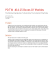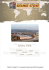# Name: Math 1314 Homework Passport 1

## Transcription

Name: Math 1314 Homework Passport 1
```Name:__________________________Math 1324 Activity 17(8.2)(Due by Apr. 10)
Dear Instructor or Tutor,
These problems are designed to let my students show me what they have learned and what
they are capable of doing on their own. Please allow them to work the problems on their
own! If you would like to help them with similar problems, here are the related homework
problems: pg. 408: 7-41 odd, 49-57 odd, 61-65 odd, 79. Thanks!
Dear Student,
Don’t even think about asking for help on the problems in this activity! The recommended
homework problems have answers in the book, so do them first for practice!!!
Two fair dice are rolled. Find the probabilities of rolling the given sums.(1-9)
First die
Second die
1,1
 2,1
 3,1
 4,1
 5,1
 6,1
1,2 
 2,2 
 3,2 
 4,2 
 5,2 
 6,2 
1,3
 2,3
 3,3
 4,3
 5,3
 6,3
1,4 
 2,4 
 3,4 
 4,4 
 5,4 
 6,4 
1,5
 2,5
 3,5
 4,5
 5,5
 6,5
1,6 
 2,6 
 3,6 
 4,6 
 5,6 
 6,6 
1. 2
2. 4
3. 5
4. 6
5. 9 or more
6. Less than 7
7. between 5 and 8(exclusive)
8. an even number
9. a multiple of 3
Tammie goes shopping and sees three kinds of shoes: flats, 2” heels, and 3” heels. The shoes
come in 2 shades of beige(light and dark) and black. If each option has an equal chance of
being selected, find the probabilities of the following events.(10-12)
Flat,light Flat,dark Flat,black
2”,light
2”,dark
2”,black
3”,light
3”,dark
3”,black
10. the shoes have a heel
11. the shoes are black
12. the shoes have a heel and are beige
Suppose that P  E   .3 , P  F   .51 , and P  E
13. Complete the following probability diagram:
E
F   .19 .(13-17)
F
S
14. P  E
F
15. P  E
F
16. P  E
F 
17. P  E
F 
Determine if the following probability assignments are valid or not, for the sample space
S  s1, s2 , s3 , s4 , s5 Tell why.(18-20)
18.
Outcome
s1
s2
s3
s4
s5
Probability .09 .32 .21 .25 .13
19.
Outcome
Probability
20.
Outcome
Probability
s1
s2
s3
s4
s5
1
3
1
4
1
6
1
8
1
10
s1
.64
s2
-.08
s3
.30
s4
.12
s5
.02
Bonus#1: What is the value of x if 820,000  820,000  2x ?
Bonus#2: A horse is tethered by a rope to a corner on the outside of a square corral that is 10
feet on each side. The horse can graze at a distance of 18 feet from the corner of the
corral where the rope is tied. What is the exact total grazing area for the horse?
Bonus#3: Given an old dog, a young boy, a string, and a tin can, what’s the
probability that the can will get tied to the dog’s tail?
Match your answer to the following probability questions with the correct lettered answers below, and place the
letter in the corresponding box at the bottom to get the answer to the riddle.
A box contains 2 blue, 3 green, and 10 red marbles. One marble is drawn at random from the box.
1) What is the probability that the marble is green?
2) What is the probability that the marble is not red?
3) What is the probability that the marble is white?
4) What is the probability that the marble is blue or green or red?
In a class of 4 boys and 6 girls, each writes his/her name on a piece of paper and puts it into a hat. Two
pieces of paper are randomly drawn from the hat without replacement.
5) What is the probability that both are girls’?
6) What is the probability that one is a boy and one is a girl?
In a class of 4 boys and 6 girls, each writes his/her name on a piece of paper and puts it into a hat. Two
pieces of paper are randomly drawn from the hat with replacement.
7) What is the probability that both are girls’?
8) What is the probability that one is a boy and one is a girl?
Two fair dice are tossed.
9) What is the probability that the sum is 7?
10) What is the probability of not getting a double?
11) What is the probability of a sum of seven or getting a double?
12) What is the probability that the sum is less than 5?
13) What is the probability that the sum is less than 13?
Three fair coins are tossed.
14) What is the probability of getting two heads and one tail?
15) What is the probability of getting one head and two tails?
Three married couples are in a room, and two people are chosen at random without replacement.
16) What is the probability that one is a male and one is a female?
17) What is the probability that both are male?
18) What is the probability that the two people are married to each other?
19) What is the probability that the two people are not married to each other?
20) What is the probability that the two people are either of the same gender or opposite gender?
9
25
1
30
8
15
B.
K.
S.
A. 0
I. 53
R. 54
C.
L.
T.
12
25
2
15
1
3
D. 56
M. 361
U. 16
E. 83
N. 1
V. 17
‘
16
5
F. 18
O. 15
W. 18
35
G. 351
P. 19
Y. 12
,
6
17
4
9
20
14
6
16
13
8
15
‘
16
2
6
7
18
10
11
1
3
8
12
19
```

### 15% OFF 2013 Lakefest Dragboat Races!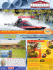### SCIOTO SHOE MART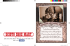### Vallelunga - Calacatta PIB (Aug 2015)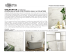### New Shoes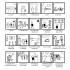### PE Happenings - September 2016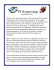### Property Brochure Template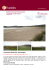### Barefoot Coaching flyer.pages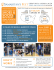### - JANUS et Cie